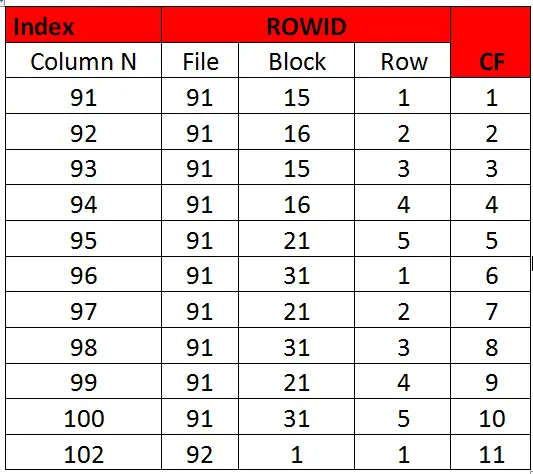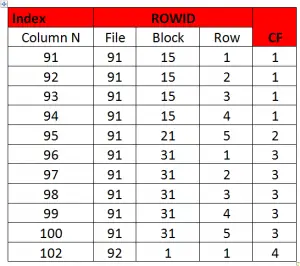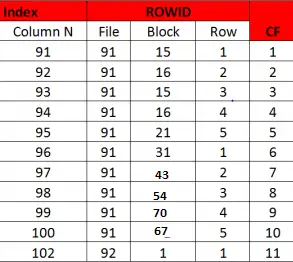# How Oracle Index clustering factor is calculatedLast updated on September 18th, 2016 at 04:51 am

### What is Oracle index clustering factor(CF)?

The clustering factor is a number which represent the degree to which data is randomly distributed in a table as compared to the indexed column. In simple terms it is the number of “block switches” while reading a table using an index.

It is an important statistics which play important role in optimizer calculation.It is used to weight the calculation for index range scan.When the clustering factor is higher, the cost of index  range scan is higher

A good Clustering factor is equal (or near) to the values of number of blocks of table.

A bad Clustering factor is equal (or near) to the number of rows of table.

### How is CF calculated?

Oracle calculates clustering factor by doing a full scan of the index walking the leaf blocks from end to end. For each entry in each leaf, Oracle checks the absolute file number and the block id, as obtained from the indexed value’s ROWID. It keeps a running count of how many “different” blocks contain data rows pointed to by the index. The block address from the first entry is compared to the block address from the second entry. If it is the same table block, Oracle does not increment the counter. If the table blocks are different, Oracle adds one to the count. This counting process continues from entry to entry always comparing the previous entry to the current one.The above is good CF example as CF is equal to number of blocks

Example of Bad Clustering factor

Here the clustering factor is equal to no of rowsThis method of counting has it own unexpected outcome. Suppose the data happens to be populated over a small set of blocks, but not in order in reference to the index key, then a set of rows could appear to be over a large set of blocks when maybe there are only a few true distinct blocks. So CF would be higher but in fact it is touching very few block.  This problem can be mitigated in 12c by using table preferences and specifying the tabled cached block.

### how to improve clustering factor in oracle

An index rebuild would not have any effect on the clustering factor. The table need to be sorted  and rebuild in order to lower the clustering factor.

### Query to determine the clustering factor

CF is stored in data dictionary and can be viewed from dba_indexes (or user_indexes).

Infact all the index statistics can be found there

SELECT index_name, index_type, uniqueness, blevel, leaf_blocks, distinct_keys, avg_leaf_blocks_per_key,
avg_data_blocks_per_key, clustering_factor, num_rows, sample_size, last_analyzed, partitioned
FROM dba_indexes
WHERE table_name = ‘ORDERS’ ;

### How Oracle index clustering factor impact the optimizer plan?

Clustering factor is the primary statistic the optimizer uses to weight index access paths. It is an estimate of the number of LIOs to table blocks required to acquire all the rows that satisfy the query in order. The higher the clustering factor, the more LIOs the optimizer will estimate will be required. The more LIOs that are required, the less attractive, and thus more costly, the use of the index will be.

## 2 thoughts on “How Oracle Index clustering factor is calculated”

1.Lakshmikant Chaudhari

Excellent….

1.techgoeasy Post Author

Thanks